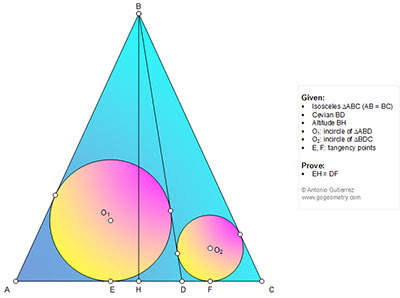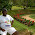## Thursday, September 24, 2015

### Geometry Problem 1149: Isosceles Triangle, Altitude, Cevian, Incircles, Tangent, Congruence

Geometry Problem. Post your solution in the comments box below.
Level: Mathematics Education, High School, Honors Geometry, College.

Click the diagram below for more details.1.Let 2.p1 and 2.p2 are perimeters of triangles ABD and BDC.
Since ABC is isosceles triangle => 2.p1- 2.p2= DA-DC= HA+HD-(HC-HD)= 2. HD
Since HA=HC => p1-p2= HD
We have DE-DF= ( p1-BA)-(p2-BC)= p1-p2 since BA=BC
So DE-DF= p1-p2= HD =HD+HE-DF = > HE=DF

2.Let the other 2 tangential points of O1 be P for AB and Q for BD. Similarly for O2, R for BD and S for AC.

Let EH = x, DF = y and FC = z

Now AE = AP = b/2 - x where AC = b
BP = BQ = a+x- b/2 where AB = BC = a
Further DF = DR and CS = CF and DE = DQ

So further since BR = BS,
(a+x-b/2) + (b/2 -y-z + x) - y = a - z

Simplifying x = y so EH = DF

Sumith Peiris
Moratuwa
Sri Lanka

3.It can further be seen that
1) < HBO1 = < DBO2
2) DO1 and DO2 are perpendicular to each other
3) AO1 and CO2 meet on BH

4.I extended the problem as follows:
http://www.fastpic.jp/images.php?file=4938134885.gif

1.We already had this problem and solution. see Problem 560 in the link below.

http://www.gogeometry.com/problem/p560_triangle_cevian_incircles_tangent_congruence.htm

Peter Tran

2.Problem 349 is another extended problem

http://gogeometry.com/problem/p349_triangle_cevian_incircles_common_tangent.htm

Thanks
Antonio